# Derivation of Biot Savart Law

The derivation of Biot Savart Law is provided in this article. Biot Savart law, named after Jean-Baptiste Biot and Felix Savart, is defined as an equation that explains the magnetic field generated by constant electric current. It plays a similar role to that of Coulomb’s law in electrostatics but in magnetostatics. Biot Savart law relates the magnetic field to magnitude, length, direction, and proximity of electric current. This law is mainly used in electromagnetism.

## Biot Savart Law Derivation

Following is the derivation of Biot Savart law.

Using electric fields that are generated by stationary charges, we get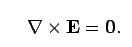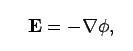Where Φ: electric scalar potential

The expression obtained from electric fields that are generated by stationary charges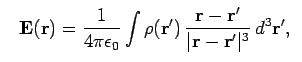Below is the Biot Savart law expression before the substitution of j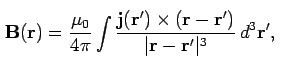Substituting for j (current density) where I is the vector current and dl is element length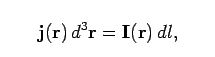After substituting for j, we obtain Biot Savart expression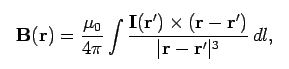### Biot Savart law for point charges

Following is the Biot Savart law derivation for point charge:

Maxwell’s equation is used for expressing electric field and magnetic field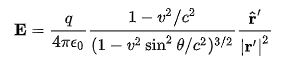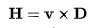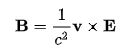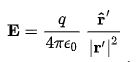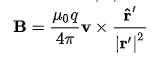Where,

q: charged particle

v: constant velocity.

Above is the equation for Biot Savart law for point charge which is derived in 1888 by Oliver Heaviside.

This was the derivation of Biot Savart law. Stay tuned with BYJU’S and learn various other Physics related topics.

Related Physics Articles:

Biot Savart law, named after Jean-Baptiste Biot and Felix Savart, is defined as an equation that explains the magnetic field generated by constant electric current. It plays a similar role to that of Coulomb’s law in electrostatics but in magnetostatics. Biot Savart law relates the magnetic field to magnitude, length, direction and proximity of electric current. This law is mainly used in electromagnetism.

## Biot Savart law derivation

Following is the derivation of Biot Savart law.

Using electric fields that are generated by stationary charges, we getWhere Φ: electric scalar potential

The expression obtained from electric fields that are generated by stationary chargesBelow is the Biot Savart law expression before the substitution of jSubstituting for j (current density) where I is the vector current and dl is element lengthAfter substituting for j, we obtain Biot Savart expression### Biot Savart law for point charges

Following is the Biot Savart law derivation for point charge:

Maxwell’s equation is used for expressing electric field and magnetic fieldWhere,

q: charged particle

v: constant velocity.

Above is the equation for Biot Savart law for point charge which is derived in 1888 by Oliver Heaviside.

This was the derivation of Biot Savart law. Stay tuned with BYJU’S and learn various other Physics related topics.

Test your knowledge on Biot-Savart law derivation